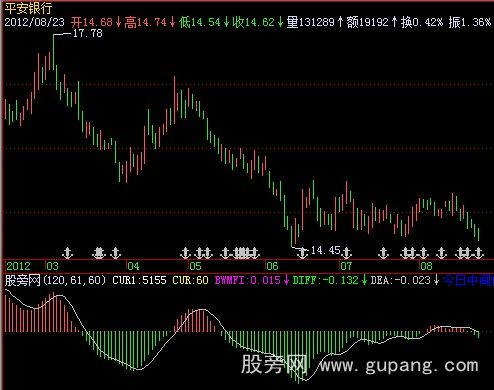# 飞狐一致性获利MACD指标公式

input:cur2(120,1,5000),cur3(61,1.5000),day1(60,1,5000);
cur1:SYSPARAM(1),linethick0;
cur:cur2-cur3+1,linethick0; //cursor 光标
BWMFI:(HIGH-LOW)*10000/VOL,linethick0;//市场促进指数
n1:=day1;
k:=day1/60;
day2:=round(5*k);
day3:=round(34*k);
TS:=BARSCOUNT(C);
V1:=(H+L)/2;
{www.gupang.com 股旁网_飞狐股票指标公式}
m5:=IF(TS<6,SUM(V1,0)/TS,MA(v1,day2));
m34:=IF(TS<35,SUM(V1,0)/TS,MA(v1,day3));
diff:m5-m34,colorstick;
DEA:IF(TS<6,SUM(DIFF,0)/TS,MA(DIFF,5));

k:=(h-l)/3;
a1:=h-k;
b1:=l+k;
if(a1<=o and o<=h and a1<=c and c<=h,11,0)linethick0;
if(l<=l and o<=b1 and l<=c and c<=b1,33,0),linethick0;
if(b1<=o and o<=a1 and b1<=c and c<=a1,22,0),linethick0;
if(l<=o and o<=b1 and a1<=c and c<=h,31,0),linethick0;
if(l<=o and o<=b1 and b1<=c and c<=a1,32,0),linethick0;
if(b1<=o and o<=a1 and a1<=c and c<=h,21,0),linethick0;
if(a1<=o and o<=h and l<=c and c<=b1,13,0),linethick0;
if(a1<=o and o<=h and b1<=c and c<=a1,12,0),linethick0;
if(b1<=o and o<=a1 and l<=c and c<=b1,23,0),linethick0;